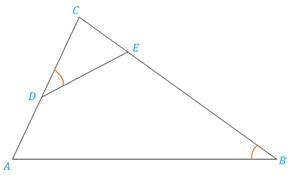Chapter 5.3, Problem 29EElementary Geometry For College St...

7th Edition
Alexander + 2 others
ISBN: 9781337614085

Solutions

Chapter
SectionElementary Geometry For College St...

7th Edition
Alexander + 2 others
ISBN: 9781337614085
Textbook Problem

Δ C D E ∼ Δ C B A with ∠ C D E ≅ ∠ B . If C D = 10 , D A = 8 and C E = 6 , find EB.Exercises 29, 30

To determine

To find:

The side EB by using the given information.

Explanation

Definition:

CSSTP:

Corresponding sides of similar triangles are proportional.

Description:

Given that ΔCDEΔCBA with CDEB. If CD=10,DA=8 and CE=6.

The given figure is shown below.

Figure 1

From the given figure, it is observed that there are two triangles CDE and CBA and which is given that two triangles are similar.

Now draw the two triangles separately as shown below.

Figure 2

Figure 3

Since ΔCDEΔCBA and from the definition of CSSTP, corresponding sides of similar triangles are proportional.

That is, CDCB=CECA=DEBA.

It is given that CD=10,DA=8 and CE=6.

Find the side EB.

Consider the first two pairs of proportions CDCB=CECA in order to find EB.

From Figure 1, it is observed that CA=CD+DA

Still sussing out bartleby?

Check out a sample textbook solution.

See a sample solution

The Solution to Your Study Problems

Bartleby provides explanations to thousands of textbook problems written by our experts, many with advanced degrees!

Get Started

Sketch the graphs of the equations in Exercises 512. xy=2x3+1

Finite Mathematics and Applied Calculus (MindTap Course List)

Find the domain of the function. 34. g(t)=3t2+t

Single Variable Calculus: Early Transcendentals, Volume I

Write the sum in sigma notation. 19. x+x2+x3++xn

Single Variable Calculus: Early Transcendentals

ex(ex)2 = a) ex3 b) e3x c) 3ex d) e4x

Study Guide for Stewart's Single Variable Calculus: Early Transcendentals, 8th